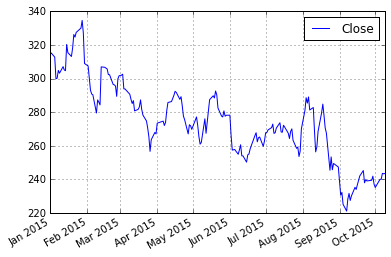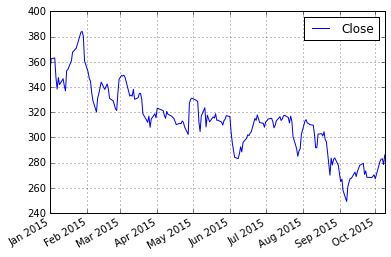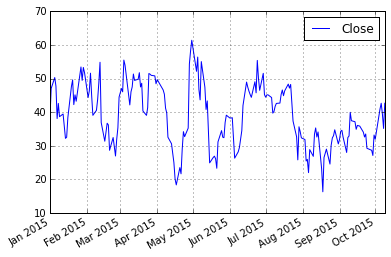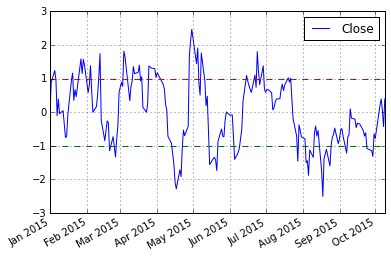In :
import numpy as np
import pandas as pd

import statsmodels
from statsmodels.tsa.stattools import coint

import matplotlib.pyplot as plt
from nsepy.archives import get_price_history
from datetime import date

In :
S1 = get_price_history(stock = 'SBIN',
start = date(2015,1,1),
end = date(2015,10,10))

S2 = get_price_history(stock = 'ICICIBANK',
start = date(2015,1,1),
end = date(2015,10,10))

result = coint(S1[['Close']], S2[['Close']])
score = result
pvalue = result

In :
S1[['Close']].plot()

Out:
<matplotlib.axes._subplots.AxesSubplot at 0x19d17400>In :
S2[['Close']].plot()

Out:
<matplotlib.axes._subplots.AxesSubplot at 0x1a29c358>In :
score, pvalue, _ = coint(S1[['Close']], S2[['Close']])
pvalue

Out:
0.0054584402716296139

In :
diff_series= S2[['Close']] - S1[['Close']]
diff_series.plot()

Out:
<matplotlib.axes._subplots.AxesSubplot at 0x19edbb38>In :
def zscore(series):
return (series - series.mean()) / np.std(series)

In :
zscore(diff_series).plot()
#plt.axhline(zscore(diff_series).mean(), color='black')
plt.axhline(1.0, color='red', linestyle='--')
plt.axhline(-1.0, color='green', linestyle='--')

Out:
<matplotlib.lines.Line2D at 0x1a7427f0>##### Simple Strategy:¶

Go "Long" the spread whenever the z-score is below -1.0
Go "Short" the spread when the z-score is above 1.0
Exit positions when the z-score approaches zero

Since we originally defined the "spread" as S1-S2, "Long" the spread would mean "Buy 1 share of S1, and Sell Short 1 share of S2" (and vice versa if you were going "Short" the spread)

In :